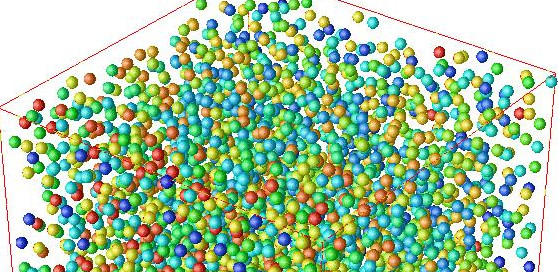# Parallel Scientific Computing I, 2021This course aims to introduce scientific computing techniques for the numerical solution of problems in science and engineering, as well as covering performance, debugging and parallel computing.

The lecture will cover:

• Fundamentals of numerical algorithms for ordinary differential equations.
• Explicit time discretion techniques for ordinary differential equations.
• Notions of error and stability analysis.
• Approaches to programming for multiple processing units using shared memory.
• Data parallel programming paradigms